# verde.BlockReduce¶

class verde.BlockReduce(reduction, spacing, region=None, adjust='spacing', center_coordinates=False)[source]

Apply a reduction/aggregation operation to the data in blocks/windows.

Returns the reduced data value for each block along with the associated coordinates, which can be determined through the same reduction applied to the coordinates or as the center of each block.

If a data region to be divided into blocks is not given, it will be the bounding region of the data. When using this class to decimate data before gridding, it’s best to use the same region and spacing as the desired grid.

If the given region is not divisible by the spacing (block size), either the region or the spacing will have to be adjusted. By default, the spacing will be rounded to the nearest multiple. Optionally, the East and North boundaries of the region can be adjusted to fit the exact spacing given.

Blocks without any data are omitted from the output.

Implements the filter method so it can be used with verde.Chain. Only acts during data fitting and is ignored during prediction.

Parameters: reduction : function A reduction function that takes an array and returns a single value (e.g., np.mean, np.median, etc). spacing : float, tuple = (s_north, s_east), or None The block size in the South-North and West-East directions, respectively. A single value means that the size is equal in both directions. region : list = [W, E, S, N] The boundaries of a given region in Cartesian or geographic coordinates. adjust : {‘spacing’, ‘region’} Whether to adjust the spacing or the region if required. Ignored if shape is given instead of spacing. Defaults to adjusting the spacing. center_coordinates : bool If True, then the returned coordinates correspond to the center of each block. Otherwise, the coordinates are calculated by applying the same reduction operation to the input coordinates.

block_split
Split a region into blocks and label points accordingly.
BlockMean
Apply the mean in blocks. Will output weights.
verde.Chain
Apply filter operations successively on data.

Methods

 filter(coordinates, data[, weights]) Apply the blocked aggregation to the given data. get_params([deep]) Get parameters for this estimator. set_params(**params) Set the parameters of this estimator.

## Examples using verde.BlockReduce¶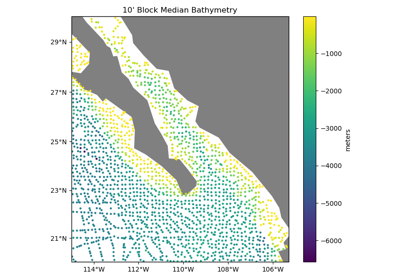Blocked reduction operations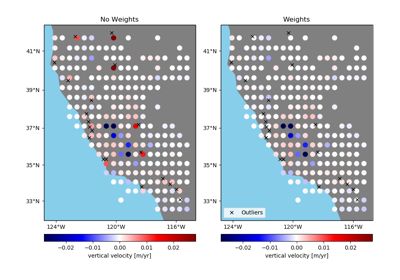Using weights in blocked reduction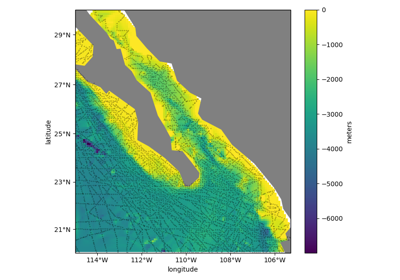Gridding with Scipy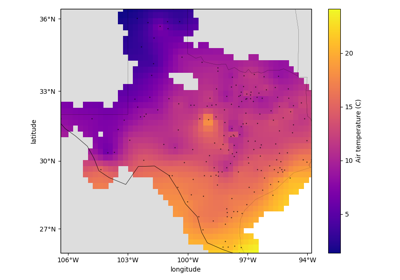Gridding with splines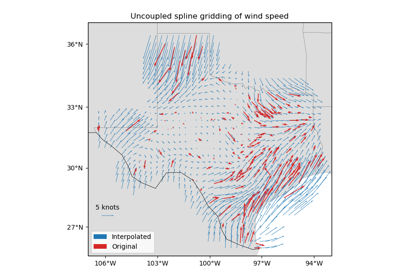Gridding 2D vectors (uncoupled)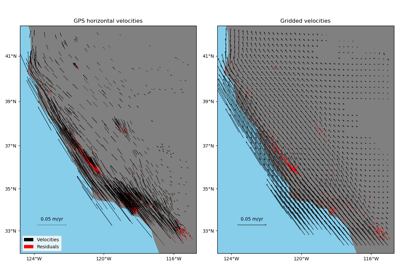Gridding 2D vectors (coupled)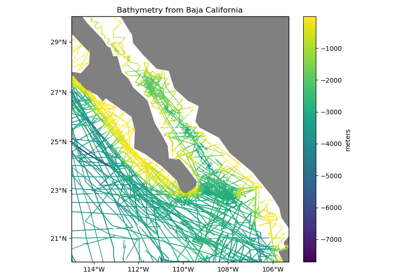Chaining Operations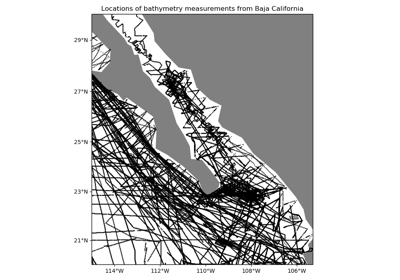Data Decimation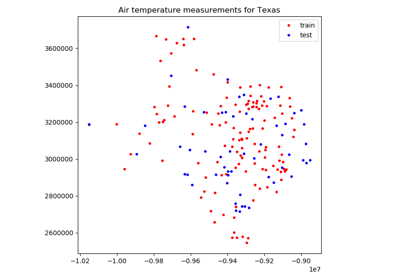Model Selection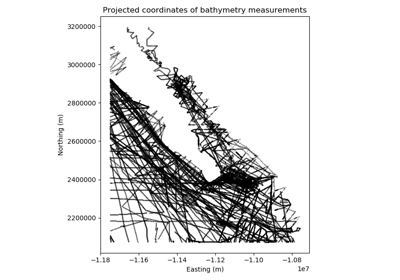Geographic Coordinates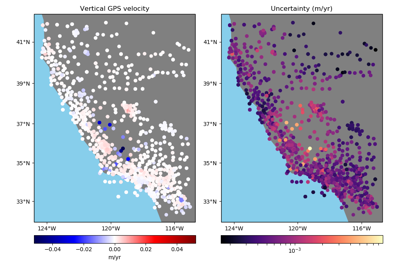Using Weights

BlockReduce.filter(coordinates, data, weights=None)[source]

Apply the blocked aggregation to the given data.

Returns the reduced data value for each block along with the associated coordinates, which can be determined through the same reduction applied to the coordinates or as the center of each block.

If weights are given, the reduction function must accept a weights keyword argument. The weights are passed in to the reduction but we have no generic way aggregating the weights or reporting uncertainties. For that, look to the specialized classes like verde.BlockMean.

Parameters: coordinates : tuple of arrays Arrays with the coordinates of each data point. Should be in the following order: (easting, northing, vertical, …). Only easting and northing will be used, all subsequent coordinates will be ignored. data : array or tuple of arrays The data values at each point. If you want to reduce more than one data component, pass in multiple arrays as elements of a tuple. All arrays must have the same shape. weights : None or array or tuple of arrays If not None, then the weights assigned to each data point. If more than one data component is provided, you must provide a weights array for each data component (if not None). blocked_coordinates : tuple of arrays (easting, northing) arrays with the coordinates of each block that contains data. blocked_data : array The block reduced data values.
BlockReduce.get_params(deep=True)

Get parameters for this estimator.

Parameters: deep : boolean, optional If True, will return the parameters for this estimator and contained subobjects that are estimators. params : mapping of string to any Parameter names mapped to their values.
BlockReduce.set_params(**params)

Set the parameters of this estimator.

The method works on simple estimators as well as on nested objects (such as pipelines). The latter have parameters of the form <component>__<parameter> so that it’s possible to update each component of a nested object.

Returns: self